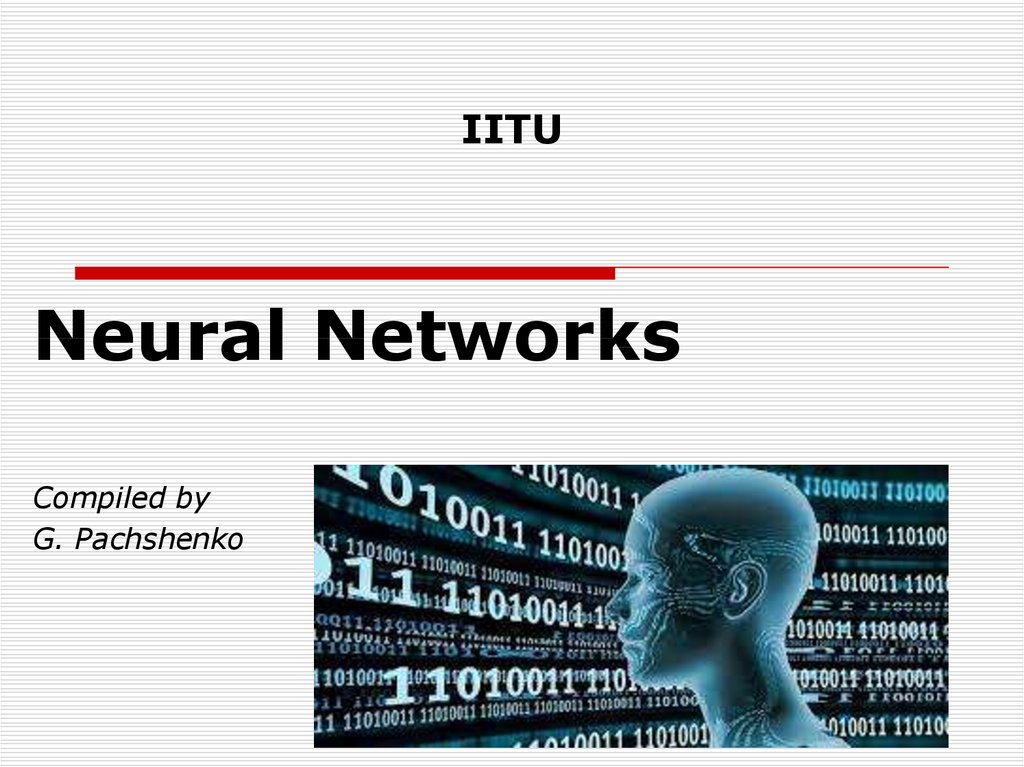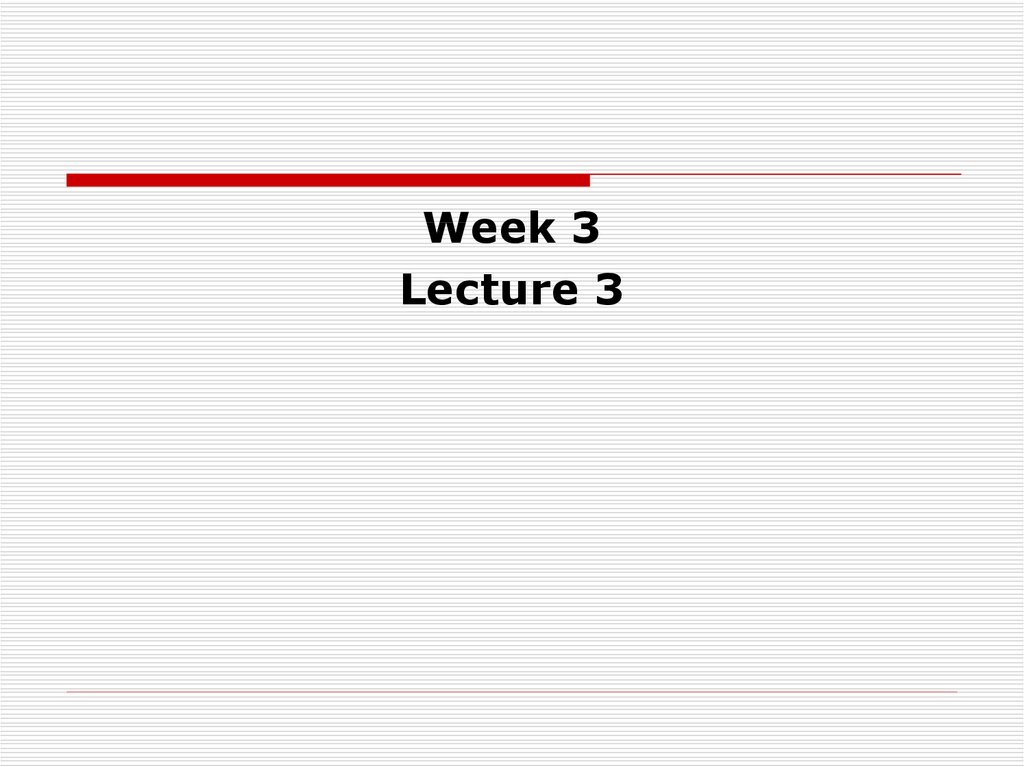# Neural Networks

Neural Networks
Compiled by
G. Pachshenko

## 2.

Pachshenko
Galina Nikolaevna
Associate Professor
of Information System
Department,
Candidate of

Week 3
Lecture 3

## 4. Topics

Perceptron
The perceptron learning algorithm
Major components of a perceptron
AND operator
OR operator
Neural Network Learning Rules
Hebbian Learning Rule

## 5.

Machine Learning Classics:
The Perceptron

## 6. Perceptron (Frank Rosenblatt, 1957)

First learning algorithm for neural
networks;
Originally introduced for character
classification, where each character is
represented as an image;

## 7.

In machine learning, the perceptron is
an algorithm for supervised
learning of binary classifiers (functions
that can decide whether an input,
represented by a vector of numbers,
belongs to some specific class or not.

## 8.

The binary classifier defines that there
should be only two categories for
classification.

## 9.

Classification is an example of
supervised learning.

## 10. The perceptron learning algorithm (PLA)

The learning algorithm for the
perceptron is online, meaning that
instead of considering the entire data
set at the same time, it only looks at
one example at a time, processes it and
goes on to the next one.

## 11.

Following are the major components of
a perceptron:

## 12.

Input: All the features become the
input for a perceptron. We denote the
input of a perceptron by [x1, x2, x3,
..,xn], where x represents the feature
value and n represents the total number
of features. We also have special kind of
input called the bias. In the image, we
have described the value of the BIAS as
w0.

## 13.

Weights: The values that are computed
over the time of training the model.
Initially, we start the value of weights
with some initial value and these values
get updated for each training error. We
represent the weights for perceptron by
[w1,w2,w3,.. wn].

## 14.

Weighted summation: Weighted
summation is the sum of the values that
we get after the multiplication of each
weight [wn] associated with the each
feature value [xn].
We represent the weighted summation
by ∑wixi for all i -> [1 to n].

## 15.

Bias: A bias neuron allows a classifier
to shift the decision boundary left or
right. In algebraic terms, the bias
neuron allows a classifier to translate its
decision boundary. It aims to "move
every point a constant distance in a
specified direction." Bias helps to train
the model faster and with better quality.

## 16.

Step/activation function: The role of
activation functions is to make neural
networks nonlinear. For
linear classification, for example, it
becomes necessary to make the
perceptron as linear as possible.

## 17.

Output: The weighted summation is
passed to the step/activation function
and whatever value we get after
computation is our predicted output.

Inputs:
1 or 0

Outputs:
1 or 0

## 20. Description:

Firstly, the features for an example are given
as input to the perceptron.
These input features get multiplied by
corresponding weights (starting with initial
value).
The summation is computed for the value we
get after multiplication of each feature with the
corresponding weight.
The value of the summation is added to the
bias.
The step/activation function is applied to the
new value.

## 23. Perceptron: Learning Algorithm

The algorithm proceeds as follows:
Initial random setting of weights;
The input is a random sequence.
For each element of class C1, if output
= 1 (correct) do nothing, otherwise
update weights;
For each element of class C2, if output
= 0 (correct) do nothing, otherwise
update weights.

## 25. Perceptron Learning Algorithm

We want to train the perceptron to
classify inputs correctly
Accomplished by adjusting the
connecting weights and the bias
Can only properly handle linearly
separable sets

## 26.

The perceptron is a machine
learning algorithm used to determine
whether an input belongs to
one class or another.
For example, the perceptron algorithm
can determine the AND operator given binary inputs and , is ( AND )
equal to 0 or 1

## 30. The AND operation between two numbers. A red dot represents one class ( AND ) and a blue dot represents the other class

The AND operation between two numbers. A red dot
represents one class ( AND ) and a blue dot represents the
other class ( AND ). The line is the result of the perceptron
algorithm, which separates all data points of one class from
those of the other.

## 36. Character classification

1 – 001001001001001
………………………………….
9 – 111101111001111
0 – 111101101101111

## 37. Neural Network Learning Rules

We know that, during ANN learning, to
change the input/output behavior, we
need to adjust the weights. Hence, a
method is required with the help of
which the weights can be modified.
These methods are called Learning
rules, which are simply algorithms or
equations.

## 38. Hebbian Learning Rule

This rule, one of the oldest and
simplest, was introduced by Donald
Hebb in his book The Organization of
Behavior in 1949.
It is a kind of feed-forward,
unsupervised learning.

## 39.

The Hebbian Learning Rule is a learning
rule that specifies how much the weight
of the connection between two units
should be increased or decreased in
proportion to the product of their
activation.

## 40. Rosenblatt’s initial perceptron rule

Rosenblatt’s initial perceptron rule is
fairly simple and can be summarized
by the following steps:
Initialize the weights to 0 or small
random numbers.
For each training sample:
Calculate the output value.
Update the weights.

## 41. Perceptron learning rule

The weight adjustment in the
perceptron learning rule is performed by
Wi+1 := wi + η(y − o)xi
where η > 0 is the learning rate, y is he
desired output,
o ∈ {0, 1} is the computed output, x is
the actual input to the neuron.

## 42.

Step 1 η > 0 is chosen, range [0,5;
0,7].
where η > 0 is the learning rate

## 43.

Step 2 Weigts are initialized at small
random values,
The running error E is set to 0

## 44.

Step 3 Training starts here.
For each element of class C1, if output
= 1 (correct) do nothing, otherwise
update weights;
For each element of class C2, if output
= 0 (correct) do nothing, otherwise
update weights.

## 45.

Step 4
Weights are updated

## 46.

Step 5 Cumulative cycle error is
computed by adding the present error
to initial error.

## 47.

Step 6
If i < N then i := i + 1 and we continue
the training by going back to Step 3,
otherwise we go to Step 7

## 48.

Step 7 The training cycle is completed.
For errow E = 0 terminate the training
session. If E > 0 then E is set to 0, N :=
1 and we initiate a new training cycle by
going to Step 3

## 49.

The output value is the class label
predicted by the unit step function that
we defined earlier.

## 50.

The value for updating the weights at
each increment is calculated by the
learning rule

## 51.

Hebbian learning rule – It identifies,
how to modify the weights of nodes of a
network.
Perceptron learning rule – Network
starts its learning by assigning a random
value to each weight.

## 52.

Thank you
for your attention!